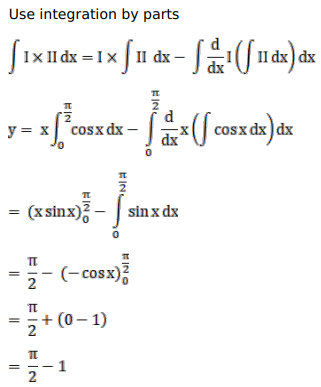# Mark against the correct answer in the following:

Question:

Mark $(\sqrt{ })$ against the correct answer in the following:

$\int_{0}^{\pi / 2} x \cos x d x=?$

A. $\frac{\pi}{2}$

B. $\left(\frac{\pi}{2}-1\right)$

C. $\left(\frac{\pi}{2}+1\right)$

D. none of these

Solution: